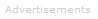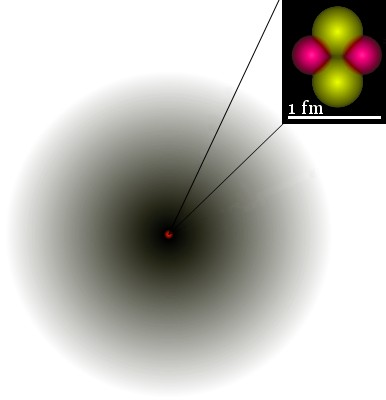# Definition of Hydrogenic

Hydrogenic means like hydrogen. Hydrogenic species are those that, like hydrogen, consist of a single nucleus orbited by a single electron.1s orbital of He+. Image by Yzmo.

Examples of hydrogenic ions are: He+, Li2+, Be3+, B4+, C5+, etc.

Mathematically, hydrogenic species are two-body systems, which are much more easily analyzed than systems involving more than two bodies.

Exact, analytic solutions of the Schrödinger equation are possible only for two-body systems; for all other atoms, ions, and molecules, no analytic solutions exist; approximation methods are utilized to solve the Schrödinger equation.

The Schrödinger equation can be used to find the wave function Ψ and its square Ψ2 for atoms, ions and molecules: analytic solutions for hydrogenic species; approximations for all others.

From Ψ and Ψ2 we get electrons' quantum numbers and the familiar shapes of atomic orbitals.

For example, the image shows the 1s orbital of He+. The probability density of the electron is indicated by the darkness of shading.

Familiar shapes of the s, p, d, and f orbitals are obtained from applying the Schrödinger equation to hydrogenic species; it turns out that these shapes are also very useful approximations in studies of non-hydrogenic species.Search the Dictionary for More Terms